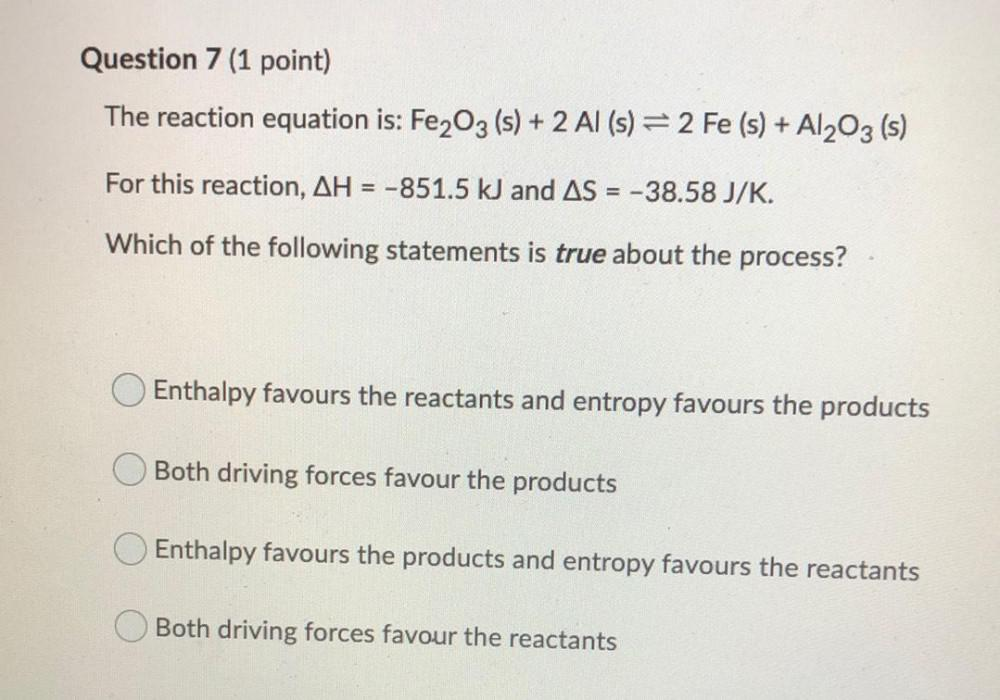Question:

# Question 7 (1 point) The reaction equation is: Fe2O3 (s) + 2 Al (s) = 2 Fe (s) + A1203 (s) For this reaction, AH = -851.5 kJ andQuestion 7 (1 point) The reaction equation is: Fe2O3 (s) + 2 Al (s) = 2 Fe (s) + A1203 (s) For this reaction, AH = -851.5 kJ and AS = -38.58 J/K. Which of the following statements is true about the process? O Enthalpy favours the reactants and entropy favours the products Both driving forces favour the products Enthalpy favours the products and entropy favours the reactants Both driving forces favour the reactants